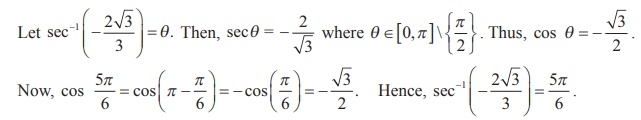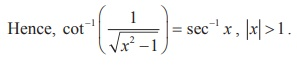Home | | Maths 12th Std | Principal Value of Inverse Trigonometric Functions

# Principal Value of Inverse Trigonometric Functions

When there are two values, one is positive and the other is negative such that they are numerically equal, then the principal value of the inverse trigonometric function is the positive one.

Principal Value of Inverse Trigonometric Functions

Let us recall that the principal value of a inverse trigonometric function at a point x is the value of the inverse function at the point x , which lies in the range of principal branch. For instance, the principal value of cos−1 (√3/2) is π/6.  Since π /6 [0, π].

When there are two values, one is positive and the other is negative such that they are numerically equal, then the principal value of the inverse trigonometric function is the positive one. Now, we list out the principal domain and range of trigonometric functions and the domain and range of inverse trigonometric functions.### Example 4.12

Find the principal value of

(i) cosec-1 (-1) (ii) sec-1 (-2) .

### Solution

(i) Let cosec-1 (-1) = y . Then, cosec y = -1

Since the range of principal value branch of y= cosec-1 x is [- π/2 , π/2] \ {0} andThus, the principal value of cosec-1 (-1) is – π/2 .

(ii) Let y = sec-1 (-2) . Then, sec y = -2 .

By definition, the range of the principal value branch of y = sec-1 x is [0,π ]\ {π /2} .

Let us find y in [0,π ] – {π/2} such that sec y = -2 .

But, sec y = −2 cos y = − 1/2 .

Now, cos y =- 1/2 = -cos π/3 = cos (π – π/3 ) = cos 2π/3 . Therefore, y = 2π/3 .

Since 2π/3 [0, π ] \ {π/2 } , the principal value of sec-1 (-2) is 2π/3 .

### Example 4.13

Find the value of sec-1(- 2√3 / 2)

### Solution### Example 4.14

If cot-1 ( 1/7 ) = θ , find the value of cos θ .

### Solution

By definition, cot−1 x (0, π) .

Therefore, cot-1 (1/7) = θ implies cot θ (0,π ) .But cot-1 ( 1/7 ) = θ implies cot θ = 1/7 and hence tan θ = 7 and θ is acute.

Using tan θ = 7/1 , we construct a right triangle as shown . Then, we have, cosθ = 1/ 5√2  .

### Example 4.15

Show that, x > 1 .

### SolutionWe construct a right triangle with the given data.

From the triangle, secα = x/1 = x . Thus, α = sec-1 x .Tags : Definition, Solved Example Problems , 12th Mathematics : UNIT 4 : Inverse Trigonometric Functions
Study Material, Lecturing Notes, Assignment, Reference, Wiki description explanation, brief detail
12th Mathematics : UNIT 4 : Inverse Trigonometric Functions : Principal Value of Inverse Trigonometric Functions | Definition, Solved Example Problems